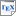[FKP10] L. Feng, M. Kwiatkowska and D. Parker. Compositional Verification of Probabilistic Systems Using Learning. In Proc. 7th International Conference on Quantitative Evaluation of Systems (QEST'10), pages 133-142, IEEE CS Press. September 2010. [pdf] [bib] Downloads:pdf (536 KB)bib Links: [Google] [Google Scholar] [CiteSeer] Abstract. We present a fully automated technique for compositional verification of probabilistic systems. Our approach builds upon a recently proposed assume-guarantee framework for probabilistic automata, in which assumptions and guarantees are probabilistic safety properties, represented using finite automata. A limitation of this work is that the assumptions need to be created manually. To overcome this, we propose a novel learning technique based on the L* algorithm, which automatically generates probabilistic assumptions using the results of queries executed by a probabilistic model checker. Learnt assumptions either establish satisfaction of the verification problem or are used to generate a probabilistic counterexample that refutes it. In the case where an assumption cannot be generated, lower and upper bounds on the probability of satisfaction are produced. We illustrate the applicability of the approach on a range of case studies.